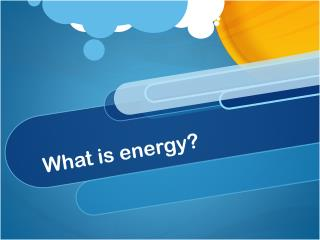DownloadDownload PresentationWhat is energy?

# What is energy?

Télécharger la présentation## What is energy?

- - - - - - - - - - - - - - - - - - - - - - - - - - - E N D - - - - - - - - - - - - - - - - - - - - - - - - - - -
##### Presentation Transcript

1. What is energy?

2. Chain of energy

3. Newton’s Laws • An object in motion will stay in motion (or rest at rest) unless acted on by an outside force. (Inertia) • Force = mass x acceleration (Units: Newtons = kg x m/s2) • For every action there is an equal and opposite reaction.

4. Work • Energy = ability to do work • Work = force exerted over a distance • W (or “energy”) = F xd • 1 Joule = 1 Newton x 1 meter • 4.18 Joule = 1.00 calorie

5. Power • Rate at which work is done • Power = Work / time • Watts = Joules / seconds • SO… Energy can also be expressed this way: • Energy = Power x time • Joules = Watts x seconds

6. Consider: • What does it mean to give electrical appliances a rating in watts? • What is a kilowatt? What is a killowatt-hour? • Why do we describe food in terms of calories?

7. Forms of Energy • Kinetic energy = Energy of moving objects • KE = ½ mass x velocity2 • KE = ½ mv2 • Joules = ½ kg x (m/s)2 • Potential energy = Energy stored due to position or composition • PE = mass x acceleration due to gravity x height • PE = mgh • Joules = kg x m/s2xm

8. Consider: • How do each of the following represent KE? • Heat radiating from a car • The sound of my voice • Muscles contracting • Light traveling to your eye

9. Consider: • How do each of the following represent potential energy? • A book on the edge of a table • A slice of pizza • Two magnets near each other • An electrical outlet • A stretched rubber band

10. What is heat? • Kinetic energy caused by atoms moving and colliding • Flows from warm body to cold body • Not the same as temperature

11. Temperature Scales • ° F = (1.8 x ° C) + 32 • ° C = (° F – 32) / 1.8 • Both use freezing and boiling points of water as standards • Kelvin = ° C – 273 • 0 K = absolute zero

12. Specific Heat • Ability of a material to absorb/lose heat • Q = mcΔt • Heat gained/lost = mass x specific heat x change in temp • If two substances have equal mass and equal change in temp., what does the amount of heat gained depend on?

13. Heat transfer • Conduction – transfer due to atoms colliding or vibrating • Convection – transfer due to bulk movement of a fluid (gas or liquid) • Radiation – transfer due to electromagnetic radiations (uses electrical/magnetic fields instead of matter) • file:///Users/johnb/Desktop/lsps07_int_heattransfer/lsps07_int_heattransfer.html • How can these types of transfer work together?

14. 1st Law of Thermodynamics:Conservation of Energy • Energy cannot be created or destroyed but can be transformed • PE = KE • mgh = ½ mv2 • How can our examples of PE be converted into KE? Is the reverse also true? • Are energy conversions 100% efficient?

15. 2nd Law of Thermodynamics: Entropy • Statements: • Heat will not flow spontaneously from a cold to a hot body. • You cannot construct an engine that does nothing but convert heat to useful work. • Every isolated system becomes more disordered with time. (Entropy) http://www.stolaf.edu/people/giannini/flashanimat/transport/diffusion.swf

16. Applications • Can any system transfer energy with 100% efficiency? What does this mean in living systems? • How do we maintain order in systems (especially living systems)? • Where does energy go? Is it truly “lost”?

17. Back to the Sun – Nuclear Fusion • High pressure and high temperature force atoms together • Mass converted to energy • Energy radiates into space • How do atoms relate to energy?